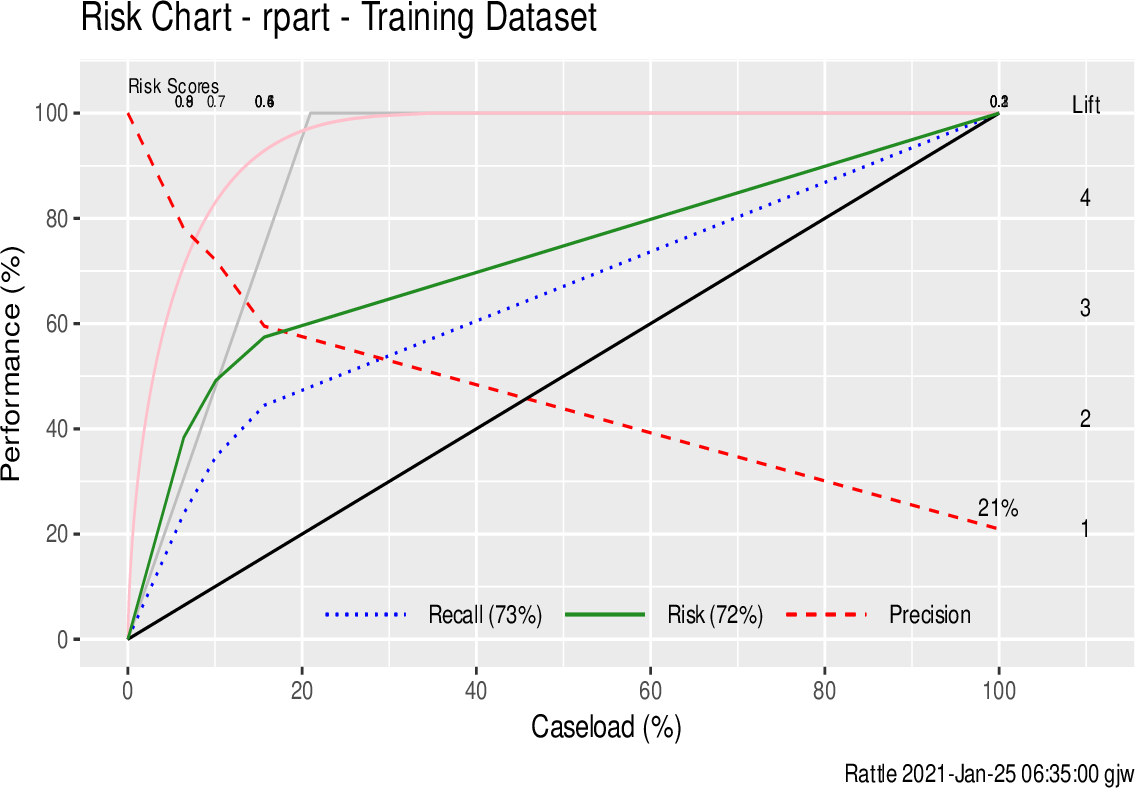Data Science Desktop Survival Guide by Graham WilliamsDesktop Survival Project Home Preface Data Science Introducing R R Constructs R Tasks R Strings R Read, Write, and Create Data Template Data Exploration Data Wrangling Data Visualisation Statistics ML Template ML Scenarios ML Activities ML Applications ML Algorithms Cluster Analysis Decision Trees Computer Vision Graph Data Privacy Literate Data Science Coding with Style Resources Bibliography Index

## Biased Estimate from the Training Dataset

We noted above that evaluating a model on the training dataset on which the model was built will result in overly optimistic performance outcomes. We can compare the performance of the randomForest::randomForest() model on the te dataset presented above with that on the training dataset. As expected the performance on the training dataset is wildly optimistic. In fact it is common for the randomForest model to predict perfectly over the training dataset as we see from the confusion matrix.

predict_tr <- predict(model, newdata=ds[tr, vars], type="class")
con(predict_tr, actual_tr)
 ```## Predicted ## Actual No Yes ## No 76 3 ## Yes 14 7 ```

Similarly Figure 12.2 illustrates the problem of evaluating a model based on the training dataset. Again we see perfect performance when we evaluate the model on the training dataset. The performance line (the Recall which is plotted as the green line) follows the best achievable which is the grey line.

pr_tr <- predict(model, newdata=ds[tr, vars], type="prob")[,2]
riskchart(pr_tr, actual_tr, risk_tr, title.size=14) +
labs(title="Risk Chart - " %s+% mtype %s+% " - Training Dataset")